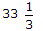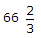# Civil Engineering - UPSC Civil Service Exam Questions

1.
Water is to be pumped at the rate of 0.025 m3/s from a 4 m deep ground level tank to an overhead tank. The difference in levels of the full tank levels of these tanks is 16 m. What is the approximate power required for operating a 65% efficient centrifugal pump ?
7.5 kW
6.0 kW
3.9 kW
3.2 kW
Explanation:
No answer description is available. Let's discuss.

2.
If in a concrete mix the fineness modulus of coarse aggregate is 7.6, the fineness modulus of fine aggregate is 2.8 and the economical value of the fineness modulus of combined aggregate is 6.4, then the proportion of fine aggregate is
25%%
50%%
Explanation:
No answer description is available. Let's discuss.

3.
A rectangular footing 1 m x 2 m is placed at a depth of 2 m in a saturated clay having an unconfined compressive strength of 100 kN/m2. According to Skempton, the net ultimate bearing capacity is
420 kN/m2
412.5 kN/m2
385 kN/m2
350 kN/m2
Explanation:
No answer description is available. Let's discuss.

4.
A floating body is in stable equilibrium
when its metacentric height is zero
when the centre of gravity of the body is below the centre of buoyancy
when its metacentre is above the centre of gravity of body
in none of the above situations